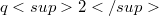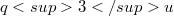﻿

### Translation planes of order$q2$ admitting collineation groups of order$q3u$ preserving a parabolic unital

#### Abstract

The set of translation planes of order$q2$ that admit collineation groups of order$q3u$, where u is a prime p-primitive divisor of$q2-1$, consists of exactly the Desarguesian plane, assuming that the group does not contain a translation subgroup of order a multiple of$q2$. This applies to show that if the group preserves a parabolic unital then the plane is forced to be Desarguesian.

DOI Code: 10.1285/i15900932v26n2p105

Keywords: Spread; Translation plane; Parabolic unital; Unital group

Classification: 51E23; 51A40

Full Text: PDF